### Fortuitous Dendrites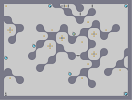Hover over the thumbnail for a full-size version.

Author ProfFiendish action author:proffiendish playable unrated 2006-08-04 4 more votes required for a rating. \$Fortuitous Dendrites#ProfFiendish#none#0001<00000000000;190000000:1000;<000000:100000000068071=00000006800000000011900000000001<00000000:=00000000000:=00000000000000000000000000000000000000;<0000000000000000000071=00000000000000;<00;19000;<00000000000:1001=00001=000000000000687900007900000000000000110000;100000000<0000001180071=000000001000007906119000000000068000;1000:10000000000001<00:180006800000000000:1000061<001<0000;<000806800001=00:=00001=0001<01<007900000000790000:=01=0;10000;<00;100000007900118007110011800000;100790611906879061<000:=0;1000:=00011000:=000000:1800000071=000000000000061<00;1900000000000000001=00:=000;<0000000000079000000001=0000000000;1000000007900000000000:=0000000;100000000000000000000711000000000000000000;19068000000|5^36,564!9^372,228,0,1,23,22,0,-1,0!9^60,180,0,0,16,1,1,-1,0!9^300,276,0,0,17,1,1,-1,0!9^564,204,0,0,20,3,1,-1,0!9^564,324,0,0,23,1,1,-1,0!9^372,36,1,1,13,20,0,0,-1!9^372,36,1,1,12,20,0,0,-1!9^372,228,0,1,23,23,0,-1,0!9^372,228,1,1,11,20,0,0,-1!9^372,36,1,0,19,13,1,0,0!11^756,564,372,228!6^756,36,1,1,0,1!6^444,204,0,1,0,0!6^36,348,1,1,0,3!6^204,276,0,1,0,3!6^300,36,0,1,0,2!6^420,444,0,1,0,0!6^756,564,0,1,0,2!0^288,276!0^300,264!0^312,276!0^300,288!0^396,36!0^552,204!0^564,192!0^576,204!0^564,216!0^564,312!0^576,324!0^564,336!0^552,324!0^72,180!0^60,168!0^60,192!0^48,180!0^372,240!0^384,228!0^372,216!0^360,228!0^492,252!0^720,420!0^636,180!0^444,204!0^528,132!0^312,180!0^60,468!0^36,36!0^264,216!0^528,384!0^492,468# I like the tileset better than the gameplay, but that may be just me.

## Other maps by this author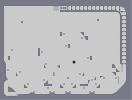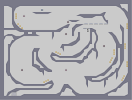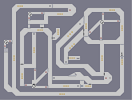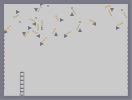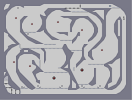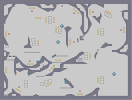Shattered Easy Shmeasy Laser Tease Floating Triangles Flowmeister Bounceblock Cave